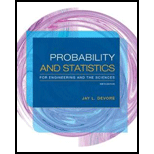# Some parts of California are particularly earthquake-prone. Suppose that in one metropolitan area. 25% of all homeowners are insured against earthquake damage. Four homeowners arc to be selected at random: let X denote the number among the four who have earthquake insurance. a. Find the probability distribution of X . [ Hint: Let S denote a homeowner who has insurance and F one who does not. Then one possible outcome is SFSS, with probability (.25)(.75)(.25)(.25) and associated X value 3. There are 15 other outcomes.] b. Draw the corresponding probability histogram. c. What is the most likely value for X ? d. What is the probability that at least two of the four selected have earthquake insurance?### Probability and Statistics for Eng...

9th Edition
Jay L. Devore
Publisher: Cengage Learning
ISBN: 9781305251809

#### Solutions

Chapter
Section### Probability and Statistics for Eng...

9th Edition
Jay L. Devore
Publisher: Cengage Learning
ISBN: 9781305251809
Chapter 3.2, Problem 16E
Textbook Problem
391 views

## Some parts of California are particularly earthquake-prone. Suppose that in one metropolitan area. 25% of all homeowners are insured against earthquake damage. Four homeowners arc to be selected at random: let X denote the number among the four who have earthquake insurance. a. Find the probability distribution of X. [Hint: Let S denote a homeowner who has insurance and F one who does not. Then one possible outcome is SFSS, with probability (.25)(.75)(.25)(.25) and associated X value 3. There are 15 other outcomes.] b. Draw the corresponding probability histogram. c. What is the most likely value for X? d. What is the probability that at least two of the four selected have earthquake insurance?

a.

To determine

Find the probability distribution of X.

### Explanation of Solution

Given info:

Some parts of California are particularly earthquake-prone. In a metropolitan area, 25% of all homeowners are insured against earthquake damage. Four home owners are selected at random. Let X be the number among the four who have earthquake insurance.

Calculation:

LetS denotes a homeowner who has insurance and F denotes a homeowner who has not insurance.

Thus,P(S)=0.25 and P(F)=0.75.

The possible values of X

X={0,ifnopersonhasinsurance.1,ifonly onepersonhasinsurance2,iftwopersonshaveinsurance3,ifthreepersonshaveinsurance4,ifallpersonshaveinsurance

The sample space is:

{FFFF,FFFS,FFSF,FSFF,SFFF,FFSS,FSFS,FSSF,SFFS,SFSF,SSFF,FSSS,SFSS,SSFS,SSSF,SSSS}

For X=0, the possible outcome is {FFFF}. That is, the frequency is 1. The probability is,

p(x)=(0.75)(0.75)(0.75)(0.75)=(0.75)4

For X=1, the possible outcome is {FFFS,FFSF,FSFF,SFFF}

b.

To determine

Draw the corresponding probability histogram.

c.

To determine

Find the most likely value for X.

d.

To determine

Find the probability that at least two of the four selected have earthquake insurance.

### Still sussing out bartleby?

Check out a sample textbook solution.

See a sample solution

#### The Solution to Your Study Problems

Bartleby provides explanations to thousands of textbook problems written by our experts, many with advanced degrees!

Get Started

Find more solutions based on key concepts
Explain two ways in which a government budget deficit hurts a future worker.

Brief Principles of Macroeconomics (MindTap Course List)

What are the techniques used to forecast human resources supply?

Foundations of Business (MindTap Course List)

Let f be the function defined by f(x) = 4x 3. Find f(4), f(14), f(0), f(a), and f(a + 1).

Applied Calculus for the Managerial, Life, and Social Sciences: A Brief Approach

Expand each expression in Exercises 122. (y1y)2

Finite Mathematics and Applied Calculus (MindTap Course List)

Find the derivatives of the functions in Problems 1-34. 30.

Mathematical Applications for the Management, Life, and Social Sciences

Evaluate the indefinite integral. 1+x1+x2dx

Single Variable Calculus: Early Transcendentals

Find a definite integral for the volume of a solid with a circular base of radius 4 inches such that the cross ...

Study Guide for Stewart's Single Variable Calculus: Early Transcendentals, 8th

True or False: By the Integral Test, converges.

Study Guide for Stewart's Multivariable Calculus, 8th